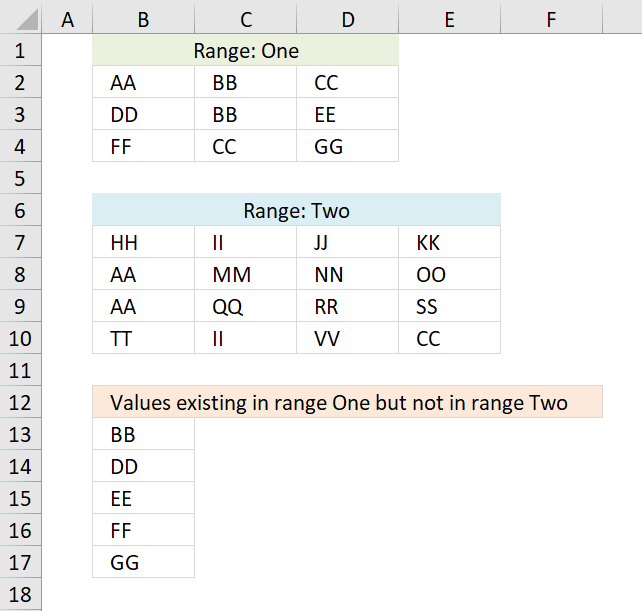Author: Oscar Cronquist Article last updated on November 27, 2018The formulas above extracts values that exists only in one or the other cell range, if you are looking for a formula that compares two separate columns the go here: Compare two columns and show differences

Array formula in B13:

=TEXTJOIN("", TRUE, IF(MIN(IF(((COUNTIF(\$B\$7:\$E\$10, \$B\$2:\$D\$4)=0)+COUNTIF(B12:\$B\$12, \$B\$2:\$D\$4))=1, (ROW(\$B\$2:\$D\$4)+(1/(COLUMN(\$B\$2:\$D\$4)+1)))*1, ""))=(ROW(\$B\$2:\$D\$4)+(1/(COLUMN(\$B\$2:\$D\$4)+1)))*1, \$B\$2:\$D\$4, ""))

Array formula in B22:

=TEXTJOIN("", TRUE, IF(MIN(IF(((COUNTIF(\$B\$2:\$D\$4, \$B\$7:\$E\$10)=0)+COUNTIF(B20:\$B\$20, \$B\$7:\$E\$10))=1, (ROW(\$B\$7:\$E\$10)+(1/(COLUMN(\$B\$7:\$E\$10)+1)))*1, ""))=(ROW(\$B\$7:\$E\$10)+(1/(COLUMN(\$B\$7:\$E\$10)+1)))*1, \$B\$7:\$E\$10, ""))

To enter an array formula, type the formula in a cell then press and hold CTRL + SHIFT simultaneously, now press Enter once. Release all keys.

The formula bar now shows the formula with a beginning and ending curly bracket telling you that you entered the formula successfully. Don't enter the curly brackets yourself.

If you don't own an Excel version that has the TEXTJOIN function then use the following considerably larger formula.

Array formula in B13:

=INDEX(\$B\$2:\$D\$4, MIN(IF((COUNTIF(\$B\$7:\$E\$10, \$B\$2:\$D\$4)=0)+COUNTIF(B12:\$B\$12, \$B\$2:\$D\$4)=1, ROW(\$B\$2:\$D\$4)-MIN(ROW(\$B\$2:\$D\$4))+1, "")), MATCH(0, COUNTIF(\$B\$7:\$E\$10, INDEX(\$B\$2:\$D\$4, MIN(IF((COUNTIF(\$B\$7:\$E\$10, \$B\$2:\$D\$4)=0)+COUNTIF(B12:\$B\$12, \$B\$2:\$D\$4)=1, ROW(\$B\$2:\$D\$4)-MIN(ROW(\$B\$2:\$D\$4))+1, "")), , 1))+COUNTIF(B12:\$B\$12, INDEX(\$B\$2:\$D\$4, MIN(IF((COUNTIF(\$B\$7:\$E\$10, \$B\$2:\$D\$4)=0)+COUNTIF(B12:\$B\$12, \$B\$2:\$D\$4)=1, ROW(\$B\$2:\$D\$4)-MIN(ROW(\$B\$2:\$D\$4))+1, "")), , 1)), 0))

copied down as far as necessary.

Formula in B21:

=INDEX(Two, MIN(IF((COUNTIF(One, Two)=0)+COUNTIF(B\$20:\$B20, Two)=1, ROW(Two)-MIN(ROW(Two))+1, "")), MATCH(0, COUNTIF(One, INDEX(Two, MIN(IF((COUNTIF(One, Two)=0)+COUNTIF(B\$20:\$B20, Two)=1, ROW(Two)-MIN(ROW(Two))+1, "")), , 1))+COUNTIF(B\$20:\$B20, INDEX(Two, MIN(IF((COUNTIF(One, Two)=0)+COUNTIF(B\$20:\$B20, Two)=1, ROW(Two)-MIN(ROW(Two))+1, "")), , 1)), 0)) + CTRL + SHIFT + ENTER

copied down as far as necessary.

### Explaining formula in cell B13

#### Step 1 - Identify values not shared by the ranges

The COUNTIF function counts values based on a condition or criteria, in this case, it counts values between the cell ranges.

COUNTIF(\$B\$7:\$E\$10, \$B\$2:\$D\$4)=0

becomes

COUNTIF({"HH", "II", "JJ", "KK";"AA", "MM", "NN", "OO";"AA", "QQ", "RR", "SS";"TT", "II", "VV", "CC"}, {"AA", "BB", "CC";"DD", "BB", "EE";"FF", "CC", "GG"})=0

becomes

{2,0,1;0,0,0;0,1,0}=0

and returns

{FALSE, TRUE, FALSE;TRUE, TRUE, TRUE;TRUE, FALSE, TRUE}.

#### Step 2 - Prevent duplicates in list

COUNTIF(B12:\$B\$12, \$B\$2:\$D\$4)

becomes

COUNTIF("Values existing in range One but not in range Two", {"AA", "BB", "CC";"DD", "BB", "EE";"FF", "CC", "GG"})

and returns

{0,0,0;0,0,0;0,0,0}.

#### Step 3 - Add arrays

Check if array is equal to 1.

((COUNTIF(\$B\$7:\$E\$10, \$B\$2:\$D\$4)=0)+COUNTIF(B12:\$B\$12, \$B\$2:\$D\$4))=1

becomes

({FALSE, TRUE, FALSE;TRUE, TRUE, TRUE;TRUE, FALSE, TRUE}+{0,0,0;0,0,0;0,0,0})=1

becomes

{0,1,0;1,1,1;1,0,1}=1

and returns

{FALSE,TRUE,FALSE;TRUE,TRUE,TRUE;TRUE,FALSE,TRUE}.

#### Step 4 - Convert TRUE to a unique number

IF(((COUNTIF(\$B\$7:\$E\$10, \$B\$2:\$D\$4)=0)+COUNTIF(B12:\$B\$12, \$B\$2:\$D\$4))=1, (ROW(\$B\$2:\$D\$4)+(1/(COLUMN(\$B\$2:\$D\$4)+1)))*1, "")

becomes

IF({FALSE, TRUE, FALSE;TRUE, TRUE, TRUE;TRUE, FALSE, TRUE}, (ROW(\$B\$2:\$D\$4)+(1/(COLUMN(\$B\$2:\$D\$4)+1)))*1, "")

becomes

IF({FALSE, TRUE, FALSE;TRUE, TRUE, TRUE;TRUE, FALSE, TRUE}, {2.33333333333333, 2.25, 2.2;3.33333333333333, 3.25, 3.2;4.33333333333333, 4.25, 4.2}, "")

and returns

{"", 2.25, "";3.33333333333333, 3.25, 3.2;4.33333333333333, "", 4.2}

#### Step 5 - Find smallest number in array

The MIN function returns the minimum number in the given array.

MIN(IF(((COUNTIF(\$B\$7:\$E\$10, \$B\$2:\$D\$4)=0)+COUNTIF(B12:\$B\$12, \$B\$2:\$D\$4))=1, (ROW(\$B\$2:\$D\$4)+(1/(COLUMN(\$B\$2:\$D\$4)+1)))*1, ""))

becomes

MIN({"", 2.25, "";3.33333333333333, 3.25, 3.2;4.33333333333333, "", 4.2})

and returns 2.25

#### Step 6 - Find position in array

IF(MIN(IF(((COUNTIF(\$B\$7:\$E\$10, \$B\$2:\$D\$4)=0)+COUNTIF(B12:\$B\$12, \$B\$2:\$D\$4))=1, (ROW(\$B\$2:\$D\$4)+(1/(COLUMN(\$B\$2:\$D\$4)+1)))*1, ""))=(ROW(\$B\$2:\$D\$4)+(1/(COLUMN(\$B\$2:\$D\$4)+1)))*1, \$B\$2:\$D\$4, "")

becomes

IF(2.25=(ROW(\$B\$2:\$D\$4)+(1/(COLUMN(\$B\$2:\$D\$4)+1)))*1, \$B\$2:\$D\$4, "")

becomes

IF(2.25={2.33333333333333, 2.25, 2.2;3.33333333333333, 3.25, 3.2;4.33333333333333, 4.25, 4.2}, \$B\$2:\$D\$4, "")

and returns {"","BB","";"","","";"","",""}.

#### Step 7 - Concatenate strings in array

The TEXTJOIN function returns values concatenated ignoring blanks in array.

TEXTJOIN("", TRUE, IF(MIN(IF(((COUNTIF(\$B\$7:\$E\$10, \$B\$2:\$D\$4)=0)+COUNTIF(B12:\$B\$12, \$B\$2:\$D\$4))=1, (ROW(\$B\$2:\$D\$4)+(1/(COLUMN(\$B\$2:\$D\$4)+1)))*1, ""))=(ROW(\$B\$2:\$D\$4)+(1/(COLUMN(\$B\$2:\$D\$4)+1)))*1, \$B\$2:\$D\$4, ""))

becomes

TEXTJOIN("", TRUE, {"","BB","";"","","";"","",""})

and returns "BB" in cell B13.

### Get Excel *.xlsx file

Filter values existing in Range 1 but not in Range 2.xlsx